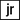# Build a Find and Replace function in Google Sheets

There are simple ways to find and replace in a spreadsheet built right into Google Sheets. And in Excel for that matter.

## What happens when we need to do it programmatically?

Javascript comes with a few methods that we can use to make this happen. The difficulty is how do we implement them in Google Apps Script.

Interacting with the data in the spreadsheet with apps script is where a lot of performance problems arise. So what we need to do is:

1. Grab the range we want from the spreadsheet
2. Read all of the values from that range
3. Manipulate that range
4. Write those values back to the spreadsheet

With this flow we are really only interacting with the spreadsheet once.

Here is how I implemented that on a spreadsheet filled with data from my Game of Thrones project a few weeks ago:

The full code is below:

``````function findAndReplace() {
//declare the range of data we are going to read

// grab all of the values from that range
var values = range.getValues();

//using the map method each parameter is all of the values in each row
var found = values.map(function(row) {
//with each row we can use reduce to process each row and return them as new rows
return row.reduce(function(acc, cell, ind) {
//if the cell is NOT a string OR the cell does NOT contain '@' we will skip it and just write the same value back into the row
if (typeof cell !== 'string' || cell.indexOf('@') === -1) {
acc[ind] = cell;
return acc;
}

//for the cells the that contain the @ symbol we take just the value to the left
acc[ind] = cell.split('@');
return acc;
}, []);
});

//finally, step 4 we write the entire array of values back to the range
range.setValues(found);
}``````

There are other ways to do this, but this is what I came up with. Check out the documentation on MDN:

Array.map on MDN

Array.reduce on MDN

If you have a better way to accomplish this, let me know in the comments below!